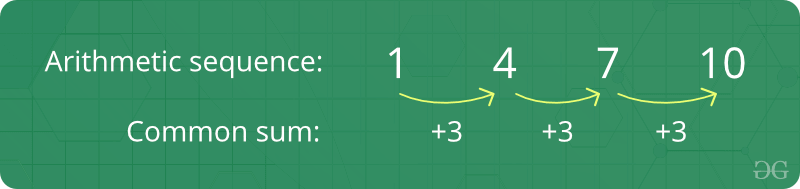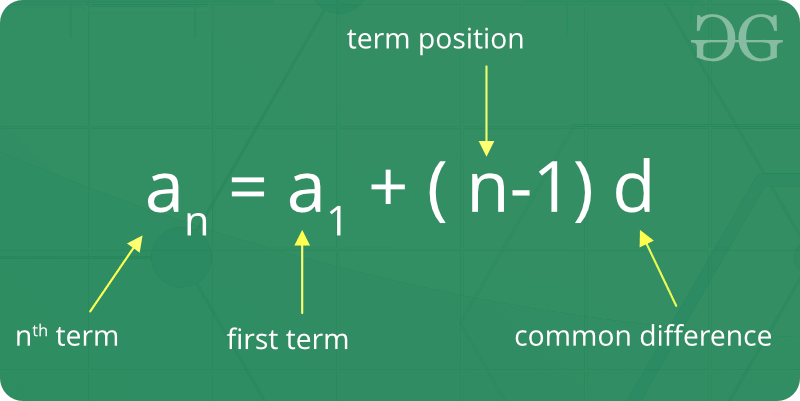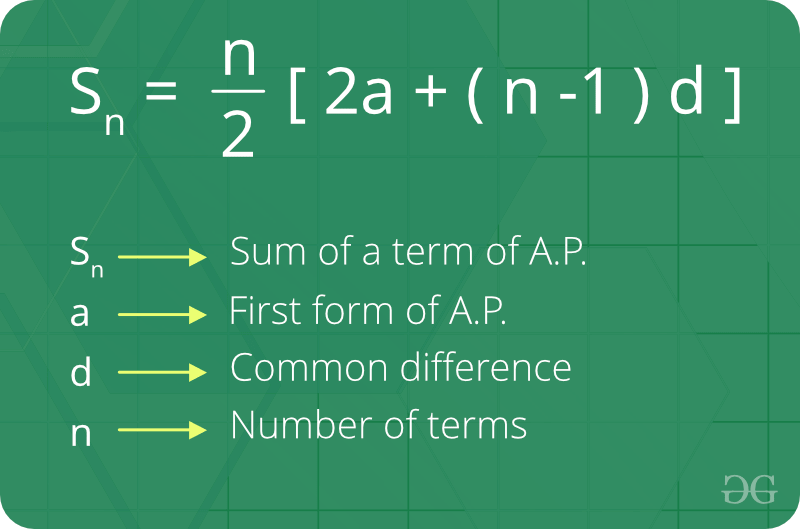Related Articles

# Arithmetic Progression

A sequence of numbers is called an Arithmetic progression if the difference between any two consecutive terms is always the same.

In simple terms, it means that the next number in the series is calculated by adding a fixed number to the previous number in the series.

Example:

Sequence 1, 4, 7,10 is an AP because the difference between any two consecutive terms in the series (common difference) is the same.

The difference between 2nd and 1st term 4 – 1 = 3
The difference between 3rd and 2nd term 7 – 4 = 3
The difference between 4th and 3rd term10 – 7 = 3From the above example, we can conclude that there is some common difference between any two consecutive elements.

## Notation in Arithmetic Progression

In AP, there are some main terms that are generally used, which are denoted as:

• Initial term (a): In an arithmetic progression, the first number in the series is called the initial term.
• Common difference (d): The value by which consecutive terms increase or decrease is called the common difference. The behavior of the arithmetic progression depends on the common difference d. If the common difference is: positive, then the members (terms) will grow towards positive infinity or negative, then the members (terms) will grow towards negative infinity.
• nth Term (an): The nth term of the AP series
• Sum of the first n terms (Sn): The sum of the first n terms of the AP series.

## The formula for the nth term of an A.P:

If ‘a’ is the initial term and ‘d’ is a common difference. Thus, the explicit formula is## The formula for the sum of n terms of AP:## How do we check whether a series is an arithmetic progression or not?

### 1. Brute approach.

The idea is to sort the given array or series. After sorting, check if the differences between consecutive elements are the same or not. If all differences are the same, Arithmetic Progression is possible.

Below is the implementation of this approach:

## C++

 `// C++ program to check if a given array` `// can form arithmetic progression` `#include ` `using` `namespace` `std;`   `// Returns true if a permutation of arr[0..n-1]` `// can form arithmetic progression` `bool` `checkIsAP(``int` `arr[], ``int` `n)` `{` `    ``if` `(n == 1)` `        ``return` `true``;`   `    ``// Sort array` `    ``sort(arr, arr + n);`   `    ``// After sorting, difference between` `    ``// consecutive elements must be same.` `    ``int` `d = arr - arr;` `    ``for` `(``int` `i = 2; i < n; i++)` `        ``if` `(arr[i] - arr[i - 1] != d)` `            ``return` `false``;`   `    ``return` `true``;` `}`   `// Driven Program` `int` `main()` `{` `    ``int` `arr[] = { 20, 15, 5, 0, 10 };` `    ``int` `n = ``sizeof``(arr) / ``sizeof``(arr);`   `    ``(checkIsAP(arr, n)) ? (cout << ``"Yes"` `<< endl) : (cout << ``"No"` `<< endl);`   `    ``return` `0;` `}`

## Java

 `// Java program to check if a given array` `// can form arithmetic progression` `import` `java.util.Arrays;`   `class` `GFG {`   `    ``// Returns true if a permutation of` `    ``// arr[0..n-1] can form arithmetic` `    ``// progression` `    ``static` `boolean` `checkIsAP(``int` `arr[], ``int` `n)` `    ``{` `        ``if` `(n == ``1``)` `            ``return` `true``;`   `        ``// Sort array` `        ``Arrays.sort(arr);`   `        ``// After sorting, difference between` `        ``// consecutive elements must be same.` `        ``int` `d = arr[``1``] - arr[``0``];` `        ``for` `(``int` `i = ``2``; i < n; i++)` `            ``if` `(arr[i] - arr[i - ``1``] != d)` `                ``return` `false``;`   `        ``return` `true``;` `    ``}`   `    ``// driver code` `    ``public` `static` `void` `main(String[] args)` `    ``{` `        ``int` `arr[] = { ``20``, ``15``, ``5``, ``0``, ``10` `};` `        ``int` `n = arr.length;`   `        ``if` `(checkIsAP(arr, n))` `            ``System.out.println(``"Yes"``);` `        ``else` `            ``System.out.println(``"No"``);` `    ``}` `}`   `// This code is contributed by Anant Agarwal.`

## Python3

 `# Python3 program to check if a given ` `# array can form arithmetic progression`   `# Returns true if a permutation of arr[0..n-1]` `# can form arithmetic progression` `def` `checkIsAP(arr, n):` `    ``if` `(n ``=``=` `1``): ``return` `True`   `    ``# Sort array` `    ``arr.sort()`   `    ``# After sorting, difference between` `    ``# consecutive elements must be same.` `    ``d ``=` `arr[``1``] ``-` `arr[``0``]` `    ``for` `i ``in` `range``(``2``, n):` `        ``if` `(arr[i] ``-` `arr[i``-``1``] !``=` `d):` `            ``return` `False`   `    ``return` `True`   `# Driver code` `arr ``=` `[ ``20``, ``15``, ``5``, ``0``, ``10` `]` `n ``=` `len``(arr)` `print``(``"Yes"``) ``if``(checkIsAP(arr, n)) ``else` `print``(``"No"``)`   `# This code is contributed by Anant Agarwal.`

## C#

 `// C# program to check if a given array` `// can form arithmetic progression` `using` `System;`   `class` `GFG {`   `    ``// Returns true if a permutation of` `    ``// arr[0..n-1] can form arithmetic` `    ``// progression` `    ``static` `bool` `checkIsAP(``int``[] arr, ``int` `n)` `    ``{` `        ``if` `(n == 1)` `            ``return` `true``;`   `        ``// Sort array` `        ``Array.Sort(arr);`   `        ``// After sorting, difference between` `        ``// consecutive elements must be same.` `        ``int` `d = arr - arr;` `        ``for` `(``int` `i = 2; i < n; i++)` `            ``if` `(arr[i] - arr[i - 1] != d)` `                ``return` `false``;`   `        ``return` `true``;` `    ``}`   `    ``// Driver Code` `    ``public` `static` `void` `Main()` `    ``{` `        ``int``[] arr = { 20, 15, 5, 0, 10 };` `        ``int` `n = arr.Length;`   `        ``if` `(checkIsAP(arr, n))` `            ``Console.WriteLine(``"Yes"``);` `        ``else` `            ``Console.WriteLine(``"No"``);` `    ``}` `}`   `// This code is contributed by vt_m.`

## PHP

 ``

## Javascript

 ``

Output

`Yes`

Time Complexity: O(n Log n).
Auxiliary Space: O(1)

### More problems related to Arithmetic Progression

Feeling lost in the world of random DSA topics, wasting time without progress? It's time for a change! Join our DSA course, where we'll guide you on an exciting journey to master DSA efficiently and on schedule.
Ready to dive in? Explore our Free Demo Content and join our DSA course, trusted by over 100,000 geeks!